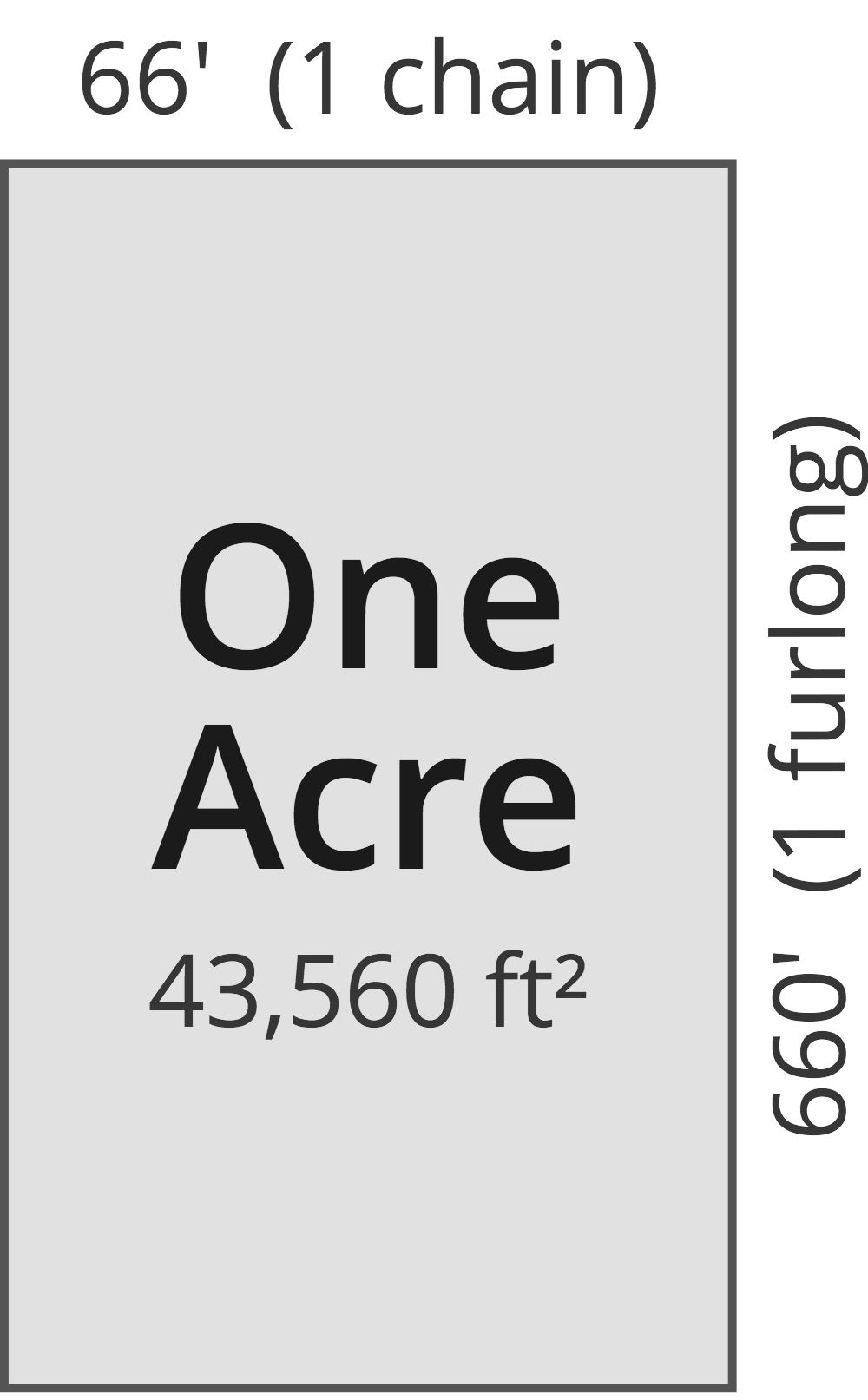# Square Yards to Acres Converter

Enter the area in square yards below to get the value converted to acres.

(find square yards)
Results in Acres:1 sq yd = 0.000207 ac

Do you want to convert acres to square yards?

## How to Convert Square Yards to Acres

To convert a measurement in square yards to a measurement in acres, divide the area by the following conversion ratio: 4,840 square yards/acre.

Since one acre is equal to 4,840 square yards, you can use this simple formula to convert:

acres = square yards ÷ 4,840

The area in acres is equal to the area in square yards divided by 4,840.

For example, here's how to convert 5,000 square yards to acres using the formula above.
acres = (5,000 sq yd ÷ 4,840) = 1.033058 ac

## What Is a Square Yard?

One square yard is equivalent to the area of a square with sides that are each 1 yard in length. One square yard is roughly equal to 9 square feet or 0.836127 square meters.

The square yard is a US customary and imperial unit of area. A square yard is sometimes also referred to as a square yd. Square yards can be abbreviated as sq yd, and are also sometimes abbreviated as yd². For example, 1 square yard can be written as 1 sq yd or 1 yd².

You can use a square yards calculator to calculate the area of a space if you know its dimensions.

## What Is an Acre?

One acre is defined as the area equal to a space that is one chain (66 ft) by one furlong (660 ft), or 10 square chains. That's equal to 43,560 square feet or 1/640 of a square mile for those unfamiliar with those units of measure.The acre is a US customary and imperial unit of area. Acres can be abbreviated as ac; for example, 1 acre can be written as 1 ac.

You can use an acreage calculator to measure the area of a plot of land in acres by locating the boundaries on a map.

## Square Yard to Acre Conversion Table

Table showing various square yard measurements converted to acres.
Square Yards Acres
1 sq yd 0.000207 ac
2 sq yd 0.000413 ac
3 sq yd 0.00062 ac
4 sq yd 0.000826 ac
5 sq yd 0.001033 ac
6 sq yd 0.00124 ac
7 sq yd 0.001446 ac
8 sq yd 0.001653 ac
9 sq yd 0.00186 ac
10 sq yd 0.002066 ac
20 sq yd 0.004132 ac
30 sq yd 0.006198 ac
40 sq yd 0.008264 ac
50 sq yd 0.010331 ac
60 sq yd 0.012397 ac
70 sq yd 0.014463 ac
80 sq yd 0.016529 ac
90 sq yd 0.018595 ac
100 sq yd 0.020661 ac
200 sq yd 0.041322 ac
300 sq yd 0.061983 ac
400 sq yd 0.082645 ac
500 sq yd 0.103306 ac
600 sq yd 0.123967 ac
700 sq yd 0.144628 ac
800 sq yd 0.165289 ac
900 sq yd 0.18595 ac
1,000 sq yd 0.206612 ac
2,000 sq yd 0.413223 ac
3,000 sq yd 0.619835 ac
4,000 sq yd 0.826446 ac
5,000 sq yd 1.0331 ac
6,000 sq yd 1.2397 ac
7,000 sq yd 1.4463 ac
8,000 sq yd 1.6529 ac
9,000 sq yd 1.8595 ac
10,000 sq yd 2.0661 ac

## References

1. National Institute of Standards and Technology, Specifications, Tolerances, and Other Technical Requirements for Weighing and Measuring Devices, Handbook 44 - 2019 Edition, https://nvlpubs.nist.gov/nistpubs/hb/2019/NIST.HB.44-2019.pdf